## How to Calculate and Solve for Corrosion Penetration Rate | Corrosion

The corrosion penetration rate is illustrated by the image below.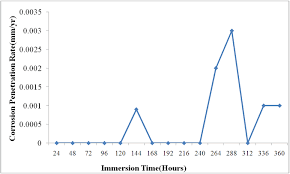To compute for corrosion penetration rate, five essential parameters are needed and these parameters are Constant (k), Weight Loss after Corrosion (W), Density of Corroding Media (ρ), Cross-sectional Area (A) and Time (t).

The formula for calculating corrosion penetration rate:

CPR = kW/ρAt

Where:

CPR = Corrosion Penetration Rate
k = Constant
W = Weight Loss after Corrosion
ρ = Density of Corroding Media
A = Cross-sectional Area
t = Time

Let’s solve an example;
Find the corrosion penetration rate when the constant is 2, the weight loss after corrosion is 4, the density of corroding media is 6, the cross-sectional area is 12 and time is 7.

This implies that;

k = Constant = 2
W = Weight Loss after Corrosion = 4
ρ = Density of Corroding Media = 6
A = Cross-sectional Area = 12
t = Time = 7

CPR = kW/ρAt
CPR = (2)(4)/(6)(12)(7)
CPR = 8/504
CPR = 0.0158

Therefore, the corrosion penetration rate is 0.0158.

## How to Calculate and Solve for Fraction of Surface Covered by Adsorbed | Mineral Processing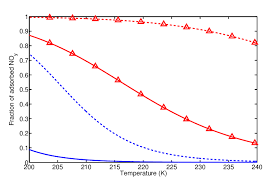The image above represents fraction of surface covered by adsorbed.

To compute for fraction of surface covered by adsorbed, two essential parameters are needed and these parameters are Constant (K) and Gas Pressure (P).

The formula for calculating the fraction of surface covered by adsorbed:

θ = KP / 1 + KP

Where:

θ = Fraction of Surface Covered by Adsorbed
K = Constant
P = Gas Pressure

Let’s solve an example;
Find the fraction of surface covered by adsorbed, given that constant is 14 and gas pressure is 24.

This implies that;

K = Constant = 14
P = Gas Pressure = 24

θ = KP / 1 + KP
θ = (14)(24) / 1 + (14)(24)
θ = 336 / 1 + 336
θ = 336 / 337
θ = 0.99

Therefore, the fraction of surface covered by adsorbed is 0.99.

## How to Calculate and Solve for Energy Used in Comminution | Mineral Processing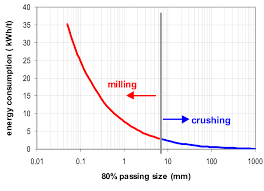The image above represents energy used in comminution.

To compute for energy used in comminution, three essential parameters are needed and these parameters are Constant (KR), Surface Area of Product (Ap) and Surface Area of Feed (Af).

The formula for calculating the energy used in comminution:

E = KR(Ap – Af)

Where:

E = Energy used in Comminution
KR = Constant
Ap = Surface Area of Product
Af = Surface Area of Feed

Let’s solve an example;
Find the energy used in comminution when the constant is 32, the surface area of product is 8 and the surface area of feed is 4.

This implies that;

KR = Constant = 32
Ap = Surface Area of Product = 8
Af = Surface Area of Feed = 4

E = KR(Ap – Af)
E = 32(8 – 4)
E = 32(4)
E = 128

Therefore, the energy used in comminution is 128 J.

Calculating the Constant when the Energy used in Comminution, the Surface Area of Product and the Surface Area of Feed is Given.

KR = E / (Ap – Af)

Where:

KR = Constant
E = Energy used in Comminution
Ap = Surface Area of Product
Af = Surface Area of Feed

Let’s solve an example;
Find the constant when the energy used in comminution is 24, the surface area of product is 15 and the surface area of feed is 7.

This implies that;

E = Energy used in Comminution = 24
Ap = Surface Area of Product = 15
Af = Surface Area of Feed = 7

KR = E / (Ap – Af)
KR = 24 / (15 – 7)
KR = 24 / 8
KR = 3

Therefore, the constant is 3.

## How to Calculate and Solve for Energy Input | Rittinger’s Equation of Comminution | Mineral Processing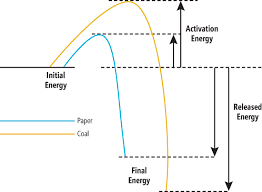The image above represents energy input.

To compute for energy input, three essential parameters are needed and these parameters are Constant (K), Feed Size (D1) and Product Size (D2).

The formula for calculating energy input:

E = K(1/D21/D1)

Where:

E = Energy Input
K = Constant
D1 = Feed Size
D2 = Product Size

Let’s solve an example;
Find the energy input when the constant is 10, the feed size is 2 and the product size is 8.

This implies that;

K = Constant = 10
D1 = Feed Size = 2
D2 = Product Size = 8

E = K(1/D21/D1)
E = 10(1/81/2)
E = 10(0.125 – 0.5)
E = 10(-0.375)
E = -3.75

Therefore, the energy input is -3.75 J.

## How to Calculate and Solve for Freezing Time | Solidification of Metals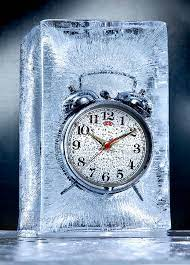The image above represents freezing time.

To compute for freezing time, three essential parameters are needed and these parameters are Thickness of Solidified Metal (M), Constant (β) and Heat Diffusivity (α).

The formula for calculating freezing time:

t = / 4β²α

Where:

t = Freezing Time
M = Thickness of Solidified Metal
β = Constant
α = Heat Diffusivity

Let’s solve an example;
Find the freezing time when the thickness of solidified metal is 8, the constant is 10 and the heat diffusivity is 2.

This implies that;

M = Thickness of Solidified Metal = 8
β = Constant = 10
α = Heat Diffusivity = 2

t = / 4β²α
t = / 4(10)²(2)
t = 64 / 4(100)(2)
t = 64 / 800
t = 0.08

Therefore, the freezing time is 0.08 s.

Calculating the Thickness of Solidified Metal when the Freezing Time, the Constant and the Heat Diffusivity is Given.

M = t x 4β²α

Where:

M = Thickness of Solidified Metal
t = Freezing Time
β = Constant
α = Heat Diffusivity

Let’s solve an example;
Find the thickness of solidified metal when the freezing time is 21, the constant is 9 and the heat diffusivity is 2.

This implies that;

t = Freezing Time = 21
β = Constant = 9
α = Heat Diffusivity = 2

M = t x 4β²α
M = 21 x 4(9²)(2)
M = 21 x 4(81)(2)
M = 21 x 4(162)
M = 21 x 648
M = 13668

Therefore, the thickness of solidified metal is 13668.

## How to Calculate and Solve for Permeability | Mass Transfer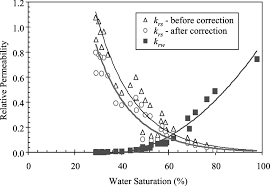The image above represents permeability.

To compute for permeability, five essential parameters are needed and these parameters are Constant (A), Partial Pressure (Po), Activation due to Permeation (Qp), Gas Constant (R) and Temperature (T).

The formula for calculating permeability:

P = APo0.5 . e-Qp/RT

Where:

P = Permeability
A = Constant
Po = Partial Pressure
Qp = Activation due to Permeation
R = Gas Constant
T = Temperature

Let’s solve an example;
Find the permeability when the constant is 12, the partial pressure is 22, the activation due to permeation is 10, the gas constant is 14 and the temperature is 2.

This implies that;

A = Constant = 12
Po = Partial Pressure = 22
Qp = Activation due to Permeation = 10
R = Gas Constant = 14
T = Temperature = 2

P = APo0.5 . e-Qp/RT
P = 12(22)0.5 . e-(10)/(14)(2)
P = 12(4.69) . e-10/28
P = 56.28 . e-0.357
P = 56.28 (0.699)
P = 39.38

Therefore, the permeability is 39.38.

## How to Calculate and Solve for Permeability due to Partial Pressure | Mass Transfer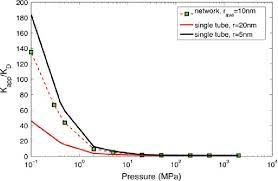The image above represents permeability due to partial pressure.

To compute for permeability due to partial pressure, three essential parameters are needed and these parameters are Diffusion Coefficient (D), Constant (K) and Partial Pressure (Po).

The formula for calculating permeability due to partial pressure:

P = DK / √(Po)

Where:

P = Permeability due to Partial Pressure
D = Diffusion Coefficient
K = Constant
Po = Partial Pressure

Let’s solve an example;
Find the permeability due to partial pressure when the diffusion coefficient is 12, the constant is 14 and the partial pressure is 17.

This implies that;

D = Diffusion Coefficient = 12
K = Constant = 14
Po = Partial Pressure = 17

P = DK / √(Po)
P = (12)(14) / √(17)
P = 168 / 4.12
P = 40.74

Therefore, the permeability due to partial pressure is 40.74.

Calculating the Diffusion Coefficient when the Permeability due to Partial Pressure, the Constant and the Partial Pressure is Given.

D = P x √(Po) / K

Where;

D = Diffusion Coefficient
P = Permeability due to Partial Pressure
K = Constant
Po = Partial Pressure

Let’s solve an example;
Find the diffusion coefficient when the permeability due to partial pressure is 12, the constant is 8 and the partial pressure is 16.

This implies that;

P = Permeability due to Partial Pressure = 12
K = Constant = 8
Po = Partial Pressure = 16

D = P x √(Po) / K
D = 12 x √(16) / 8
D = 12 x 4 / 8
D = 48 / 8
D = 6

Therefore, the diffusion constant is 6.

## How to Calculate and Solve for Diffusion Coefficient | Mass Transfer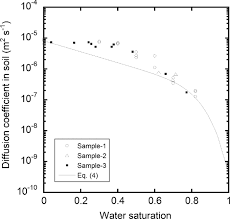The image above represents diffusion coefficient.

To compute for diffusion coefficient, three essential parameters are needed and these parameters are Constant (BA), Boltzmann’s Constant (KB) and Temperature (T).

The formula for calculating diffusion coefficient:

DA = BAKBT

Where:

DA = Diffusion Coefficient | Nernst-Einstein Equation
BA = Constant
KB = Boltzmann’s Constant
T = Temperature

Let’s solve an example;
Find the diffusion coefficient when the constant is 21, the boltzmann’ s constant is 1.39e-23 and temperature is 12.

This implies that;

BA = Constant = 21
KB = Boltzmann’s Constant = 1.3806e-23
T = Temperature = 12

DA = BAKBT
DA = (21)(1.38e-23)(12)
DA = 3.47

Therefore, the diffusion coefficient is 3.47e-21 cm²/s.

Calculating the Constant when the Diffusion Coefficient and the Temperature is Given.

BA = DA / KB x T

Where;

BA = Constant
DA = Diffusion Coefficient | Nernst-Einstein Equation
KB = Boltzmann’s Constant
T = Temperature

Let’s solve an example;
Find the constant when the diffusion coefficient is 10 and the temperature is 3.

This implies that;

DA = Diffusion Coefficient | Nernst-Einstein Equation = 10
KB = Boltzmann’s Constant = 1.3806e-23
T = Temperature = 12

BA = DA / KB x T
BA = 10 / 1.3806e-23 x 12
BA = 10 / 1.70e-9
BA = 5.88e+9

Therefore, the constant is 5.88e+9.

## How to Calculate and Solve for Concentration of Particles | Fluidization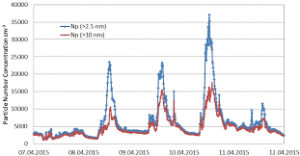The image above represents concentration of particles.

To compute for concentration of particles, three essential parameters are needed and these parameters are Initial Concentration of Particles (Co), Constant (M) and Time of Elutriation (t).

The formula for calculating concentration of particles:

C = Coe-Mt

Where:

C = Concentration of Particles
Co = Initial Concentration of Particles
M = Constant
t = Time of Elutriation

Let’s solve an example;
Find the concentration of particles when the initial concentration of particles is 10, the constant is 5 and the time of elutriation is 2.

This implies that;

Co = Initial Concentration of Particles = 10
M = Constant = 5
t = Time of Elutriation = 2

C = Coe-Mt
C = 10e-(5)(2)
C = 10e-10
C = 10(0.000045)
C = 0.00045

Therefore, the concentration of particles is 0.00045 mol/m³.

## How to Calculate and Solve for Expected Discharge | Flood Formulae | Irrigation Water Requirement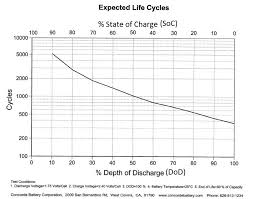The image above represents expected discharge.

To compute for expected discharge, three essential parameters are needed and these parameters are Constant (c), Constant (n) and Drainage Basin Area (Ad).

The formula for calculating expected discharge:

Where:

Qp = Expected Discharge | Flood Formulae
c = Constant
n = Constant

Let’s solve an example;
Find the expected discharge when the constant is 8, the constant is 2 and the drainage basin area is 4.

This implies that;

c = Constant = 8
n = Constant = 2
Ad = Drainage Basin Area = 4

Qp = (8) . (4)2
Qp = (8) . (16)
Qp = 128

Therefore, the expected discharge is 128.

Calculating the Constant when the Expected Discharge, the Constant and the Drainage Basin Area is Given.

Where;

c = Constant
Qp = Expected Discharge | Flood Formulae
n = Constant

Let’s solve an example;
Find the constant when the expected discharge is 30, the constant is 3 and the drainage basin area is 2.

This implies that;

Qp = Expected Discharge | Flood Formulae = 30
n = Constant = 3
Ad = Drainage Basin Area = 2